# Class 10 RD Sharma Solutions – Chapter 8 Quadratic Equations – Exercise 8.11

• Last Updated : 21 Dec, 2020

### Question 1: The perimeter of the rectangular field is 82 m and its area is 400 m2. Find the breadth of the rectangle?

Solution: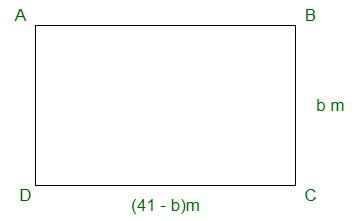Hey! Looking for some great resources suitable for young ones? You've come to the right place. Check out our self-paced courses designed for students of grades I-XII

Start with topics like Python, HTML, ML, and learn to make some games and apps all with the help of our expertly designed content! So students worry no more, because GeeksforGeeks School is now here!

Given:

Perimeter = 82 m and its area = 400 m2

Let the breadth of the rectangle be ‘b’ m.

As we know,

Perimeter of a rectangle = 2×(length + breadth)

82 = 2×(length + b)

41 = (length + b)

Length = (41 – b)m

As we know,

Area of the rectangle = length × breadth

400 = (41 – b)×(b)

400 = 41b – b2

b2 – 41b + 400 = 0

b2 – 25b – 16b + 400 = 0

b×(b – 25) – 16×(b – 25) = 0

(b – 16)(b – 25) = 0

Now,

either b – 16 = 0

⇒ b = 16

Or, b – 25 = 0

⇒ b = 25

Hence,

The breadth of the rectangle can be either 16 m or 25 m respectively.

### Question 2: The length of the hall is 5 m more than its breadth. If the area of the floor of the hall is 84 m2, what are the length and breadth of the hall?

Solution: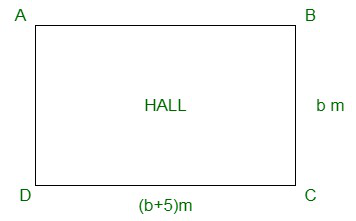Let the breadth of the rectangle be ‘b’ m

Then,

The length of the hall is 5 m more than its breadth i.e, = (b + 5) m

Given, area of the hall is = 84 m2

As the hall is rectangular,

Area of the rectangular hall = length × breadth

84 = b(b + 5)

b2 + 5b – 84 = 0

b2 + 12b – 7b – 84 = 0

b(b + 12) – 7(b + 12) = 0

(b + 12)(b – 7) = 0

Hence,

either b + 12 = 0

⇒ b = – 12 m (Side of a rectangle cannot be negative)

Or, b – 7 = 0

⇒ b = 7 m

So, only b = 7 m is considered.

⇒ b + 5 = 12

Therefore,

The length and breadth of the rectangle is 7 m and 12 m respectively.

### Question 3: Two squares have sides x and (x + 4) cm. The sum of their area is 656 cm2. Find the sides of the square.

Solution:

Let A and B be the two squares.

And, let ‘s’ cm be the side square A and (s + 4) cm be the side of the square B.

So,

Area of the square A = s2 cm2

Area of the square B =(s + 4)2 cm2

Given:

Area of the square A + Area of the square B = 656 cm2

⇒ s2 cm2 + (s + 4)2 cm2 = 656 cm2

s2+ s2 + 16 + 8s – 656 = 0

2s2 + 16 + 8s – 656 = 0

2(s2 + 4s – 320) = 0

s2 + 4s – 320 = 0

s2 + 20s – 16s – 320 = 0

s(s + 20) – 16(s + 20) = 0

(s + 20)(s – 16) = 0

Now,

either s + 20 = 0

⇒ s = -20 (Side of a square cannot be negative)

Or, s – 16 = 0 ⇒ s = 16

Hence, the value of s = 16 ⇒ s + 4 = 20

Therefore,

The side of the square A= 16 cm

The side of the square B = 20 cm

### Question 4: The area of a right-angled triangle is 165 cm2. Determine its base and altitude if the latter exceeds the former by 7m.

Solution: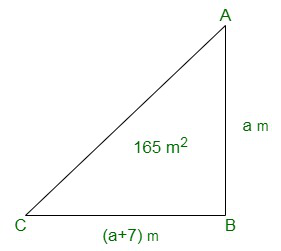Let the altitude of the right angle triangle be ‘a’ m

Given:

The altitude exceeds the base by 7m ⇒ altitude = (a – 7)m

As we know,

Area of the triangle = 1/2 × base × altitude

⇒ 165 = 1/2 × (a − 7) × a

a(a – 7) = 330

a2 – 7a – 330 = 0

a2 – 22a + 15a – 330 = 0

a(a – 22) + 15(a – 22) = 0

(a – 22)(a + 15) = 0

Now,

either a – 22 = 0 ⇒ a = 22

Or, a + 15 = 0 ⇒ a = -15 (Altitude of a triangle cannot be negative)

So the value of a = 22 is only considered

⇒ a – 7 = 15

Hence,

The base and altitude of the right angled triangle are 15 cm and 22 cm respectively.

### Question 5: Is it possible to design a rectangular mango grove whose length is twice its breadth and the area is 800 m2? If so, find its length and breadth.

Solution: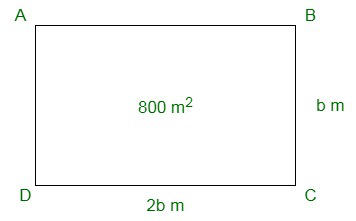Let the breadth of the rectangular mango grove be ‘b’ m

Given:

The length of rectangle is twice of its breadth.

So, length = 2b

Area of the grove = 800 m2 (given)

As we know,

Area of the rectangle = length × breadth

800 = b × (2b)

2b2 – 800 = 0

b2 – 400 = 0

⇒ b = √400 = 20 (neglecting the negative sq. root as side can never be negative)

Therefore,

The breadth of the rectangular groove is 20 m.

And, the length of the rectangular groove is 40 m.

Yes, it is possible to design a rectangular groove whose length is twice of its breadth.

### Question 6: Is it possible to design a rectangular park of perimeter 80 m and area 400 m² ? If so, find its length and breadth:

Solution: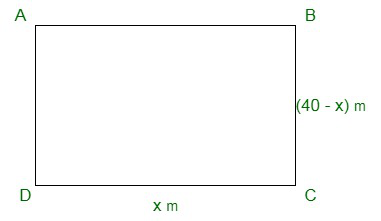Perimeter of rectangular park = 80 m

Length + Breadth = 80/2 = 40 m

Let length = x m

Them breadth = 40 – x

According to the condition,

x (40 – x) = 400

⇒ 40x – x² = 400

⇒ x² – 40x + 400 = 0

⇒ (x – 20)² = 0

⇒ x – 20 = 0

⇒ x = 20

Yes, it is possible

Length = 20 m

and breadth = 40 – x = 40 – 20 = 20 m

### Question 7: Sum of the areas of two squares is 640 m². If the difference of their perimeters is 64 m, find the sides of the two squares. [CBSE 2008]

Solution:

Let the side of the first square = x m and of second squares = y m

Given:

4x – 4y = 64

⇒ x – y = 16 ….(i)

and x² + y² = 640 ….(ii)

From (i), x = 16 + y

In (ii)

(16 + y)² + y² = 640

⇒ 256 + 32y + y² + y² = 640

⇒ 2y² + 32y + 256 – 640 = 0

⇒ y² + 16y – 192 = 0 (Dividing by 2)

⇒ y² + 24y – 8y – 192 = 0

⇒ y (y + 24) – 8 (y + 24) = 0

⇒ (y + 24)(y – 8) = 0

Either y + 24 = 0, then y = -24 (which is not possible as it is negative)

or y – 8 = 0, then y = 8

x = 16 + y = 16 + 8 = 24

Side of first square = 24 m

and side of second square = 8 m

My Personal Notes arrow_drop_up